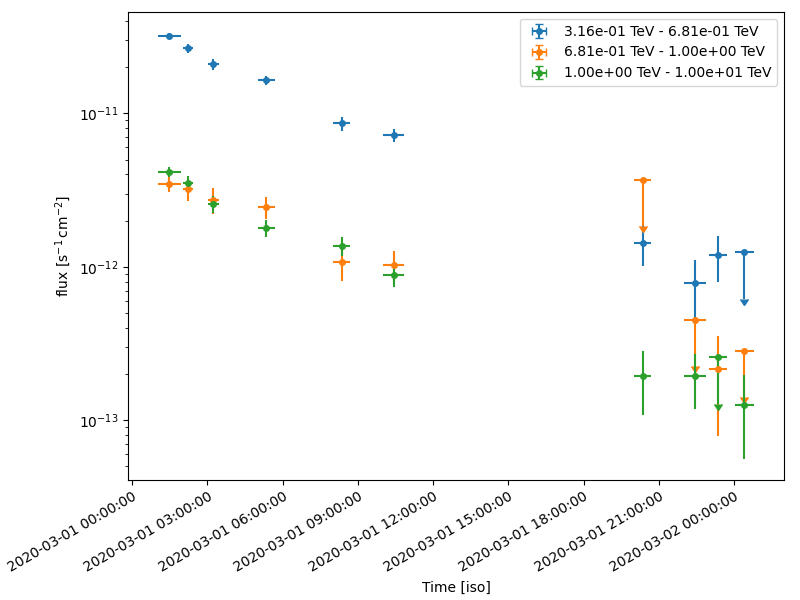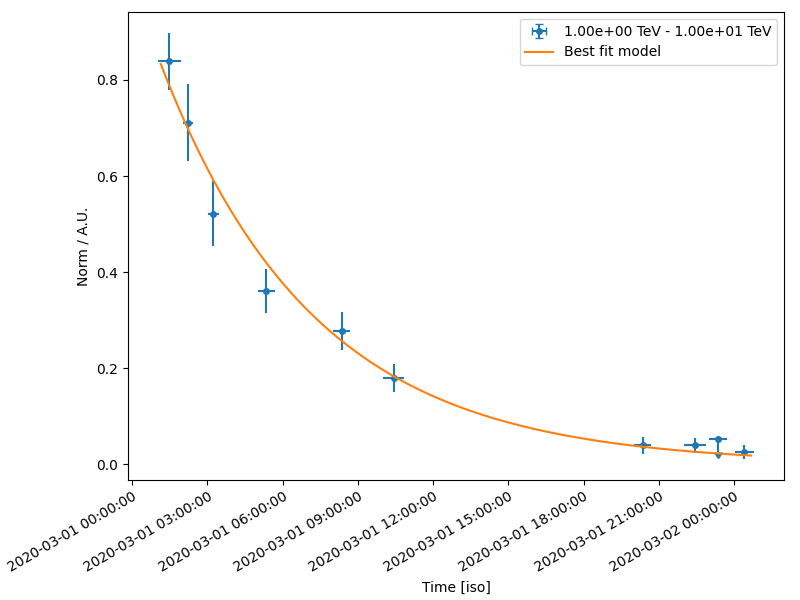# Simulating and fitting a time varying source#

Simulate and fit a time decaying light curve of a source using the CTA 1DC response.

## Context#

Frequently, studies of variable sources (eg: decaying GRB light curves, AGN flares, etc) require time variable simulations. For most use cases, generating an event list is an overkill, and it suffices to use binned simulations using a temporal model.

Objective: Simulate and fit a time decaying light curve of a source with CTA using the CTA 1DC response

## Proposed approach#

We will simulate 10 spectral datasets within given time intervals (Good Time Intervals) following a given spectral (a power law) and temporal profile (an exponential decay, with a decay time of 6 hr ). These are then analysed using the light curve estimator to obtain flux points.

Modelling and fitting of lightcurves can be done either - directly on the output of the `LighCurveEstimator` (at the DL5 level) - fit the simulated datasets (at the DL4 level)

In summary, necessary steps are:

• Choose observation parameters including a list of `gammapy.data.GTI`

• Define temporal and spectral models from :ref:model-gallery as per science case

• Perform the simulation (in 1D or 3D)

• Extract the light curve from the reduced dataset as shown in Light curves tutorial.

• Optionally, we show here how to fit the simulated datasets using a source model

## Setup#

```import logging
import numpy as np
import astropy.units as u
from astropy.coordinates import SkyCoord
from astropy.time import Time

# %matplotlib inline
import matplotlib.pyplot as plt
```

## Setup#

```from IPython.display import display

log = logging.getLogger(__name__)
```

And some gammapy specific imports

```from gammapy.data import Observation, observatory_locations
from gammapy.datasets import Datasets, FluxPointsDataset, SpectrumDataset
from gammapy.estimators import LightCurveEstimator
from gammapy.makers import SpectrumDatasetMaker
from gammapy.maps import MapAxis, RegionGeom, TimeMapAxis
from gammapy.modeling import Fit
from gammapy.modeling.models import (
ExpDecayTemporalModel,
PowerLawSpectralModel,
SkyModel,
)
```

## Check setup#

```from gammapy.utils.check import check_tutorials_setup

check_tutorials_setup()
```
```System:

python_executable      : /home/runner/work/gammapy-docs/gammapy-docs/gammapy/.tox/build_docs/bin/python
python_version         : 3.9.16
machine                : x86_64
system                 : Linux

Gammapy package:

version                : 1.1
path                   : /home/runner/work/gammapy-docs/gammapy-docs/gammapy/.tox/build_docs/lib/python3.9/site-packages/gammapy

Other packages:

numpy                  : 1.24.3
scipy                  : 1.10.1
astropy                : 5.2.2
regions                : 0.7
click                  : 8.1.3
yaml                   : 6.0
IPython                : 8.14.0
jupyterlab             : not installed
matplotlib             : 3.7.1
pandas                 : not installed
healpy                 : 1.16.2
iminuit                : 2.21.3
sherpa                 : 4.15.1
naima                  : 0.10.0
emcee                  : 3.1.4
corner                 : 2.2.2
ray                    : 2.5.0

Gammapy environment variables:

GAMMAPY_DATA           : /home/runner/work/gammapy-docs/gammapy-docs/gammapy-datasets/1.1
```

We first define our preferred time format:

```TimeMapAxis.time_format = "iso"
```

## Simulating a light curve#

We will simulate 10 spectra between 300 GeV and 10 TeV using an `PowerLawSpectralModel` and a `ExpDecayTemporalModel`. The important thing to note here is how to attach a different `GTI` to each dataset. Since we use spectrum datasets here, we will use a `RegionGeom`.

```# Loading IRFs
"\$GAMMAPY_DATA/cta-1dc/caldb/data/cta/1dc/bcf/South_z20_50h/irf_file.fits"
)

# Reconstructed and true energy axis
energy_axis = MapAxis.from_edges(
np.logspace(-0.5, 1.0, 10), unit="TeV", name="energy", interp="log"
)
energy_axis_true = MapAxis.from_edges(
np.logspace(-1.2, 2.0, 31), unit="TeV", name="energy_true", interp="log"
)

geom = RegionGeom.create("galactic;circle(0, 0, 0.11)", axes=[energy_axis])

# Pointing position
pointing = SkyCoord(0.5, 0.5, unit="deg", frame="galactic")
```
```/home/runner/work/gammapy-docs/gammapy-docs/gammapy/.tox/build_docs/lib/python3.9/site-packages/astropy/units/core.py:2097: UnitsWarning: '1/s/MeV/sr' did not parse as fits unit: Numeric factor not supported by FITS If this is meant to be a custom unit, define it with 'u.def_unit'. To have it recognized inside a file reader or other code, enable it with 'u.add_enabled_units'. For details, see https://docs.astropy.org/en/latest/units/combining_and_defining.html
warnings.warn(msg, UnitsWarning)
```

Note that observations are usually conducted in Wobble mode, in which the source is not in the center of the camera. This allows to have a symmetrical sky position from which background can be estimated.

```# Define the source model: A combination of spectral and temporal model

gti_t0 = Time("2020-03-01")
spectral_model = PowerLawSpectralModel(
index=3, amplitude="1e-11 cm-2 s-1 TeV-1", reference="1 TeV"
)
temporal_model = ExpDecayTemporalModel(t0="6 h", t_ref=gti_t0.mjd * u.d)

model_simu = SkyModel(
spectral_model=spectral_model,
temporal_model=temporal_model,
name="model-simu",
)

# Look at the model
display(model_simu.parameters.to_table())
```
```/home/runner/work/gammapy-docs/gammapy-docs/gammapy/.tox/build_docs/lib/python3.9/site-packages/astropy/units/quantity.py:673: RuntimeWarning: overflow encountered in exp
result = super().__array_ufunc__(function, method, *arrays, **kwargs)
type      name     value         unit      ... max frozen is_norm link
-------- --------- ---------- -------------- ... --- ------ ------- ----
spectral     index 3.0000e+00                ... nan  False   False
spectral amplitude 1.0000e-11 cm-2 s-1 TeV-1 ... nan  False    True
spectral reference 1.0000e+00            TeV ... nan   True   False
temporal        t0 6.0000e+00              h ... nan  False   False
temporal     t_ref 5.8909e+04              d ... nan   True   False
```

Now, define the start and observation livetime wrt to the reference time, `gti_t0`

```n_obs = 10

tstart = gti_t0 + [1, 2, 3, 5, 8, 10, 20, 22, 23, 24] * u.h
lvtm = [55, 25, 26, 40, 40, 50, 40, 52, 43, 47] * u.min
```

Now perform the simulations

```datasets = Datasets()

empty = SpectrumDataset.create(
geom=geom, energy_axis_true=energy_axis_true, name="empty"
)

maker = SpectrumDatasetMaker(selection=["exposure", "background", "edisp"])

for idx in range(n_obs):
obs = Observation.create(
pointing=pointing,
livetime=lvtm[idx],
tstart=tstart[idx],
irfs=irfs,
reference_time=gti_t0,
obs_id=idx,
location=observatory_locations["cta_south"],
)
empty_i = empty.copy(name=f"dataset-{idx}")
dataset = maker.run(empty_i, obs)
dataset.models = model_simu
dataset.fake()
datasets.append(dataset)
```
```/home/runner/work/gammapy-docs/gammapy-docs/gammapy/.tox/build_docs/lib/python3.9/site-packages/gammapy/data/observations.py:226: GammapyDeprecationWarning: Pointing will be required to be provided as FixedPointingInfo
warnings.warn(
/home/runner/work/gammapy-docs/gammapy-docs/gammapy/.tox/build_docs/lib/python3.9/site-packages/gammapy/data/observations.py:226: GammapyDeprecationWarning: Pointing will be required to be provided as FixedPointingInfo
warnings.warn(
/home/runner/work/gammapy-docs/gammapy-docs/gammapy/.tox/build_docs/lib/python3.9/site-packages/gammapy/data/observations.py:226: GammapyDeprecationWarning: Pointing will be required to be provided as FixedPointingInfo
warnings.warn(
/home/runner/work/gammapy-docs/gammapy-docs/gammapy/.tox/build_docs/lib/python3.9/site-packages/gammapy/data/observations.py:226: GammapyDeprecationWarning: Pointing will be required to be provided as FixedPointingInfo
warnings.warn(
/home/runner/work/gammapy-docs/gammapy-docs/gammapy/.tox/build_docs/lib/python3.9/site-packages/gammapy/data/observations.py:226: GammapyDeprecationWarning: Pointing will be required to be provided as FixedPointingInfo
warnings.warn(
/home/runner/work/gammapy-docs/gammapy-docs/gammapy/.tox/build_docs/lib/python3.9/site-packages/gammapy/data/observations.py:226: GammapyDeprecationWarning: Pointing will be required to be provided as FixedPointingInfo
warnings.warn(
/home/runner/work/gammapy-docs/gammapy-docs/gammapy/.tox/build_docs/lib/python3.9/site-packages/gammapy/data/observations.py:226: GammapyDeprecationWarning: Pointing will be required to be provided as FixedPointingInfo
warnings.warn(
/home/runner/work/gammapy-docs/gammapy-docs/gammapy/.tox/build_docs/lib/python3.9/site-packages/gammapy/data/observations.py:226: GammapyDeprecationWarning: Pointing will be required to be provided as FixedPointingInfo
warnings.warn(
/home/runner/work/gammapy-docs/gammapy-docs/gammapy/.tox/build_docs/lib/python3.9/site-packages/gammapy/data/observations.py:226: GammapyDeprecationWarning: Pointing will be required to be provided as FixedPointingInfo
warnings.warn(
/home/runner/work/gammapy-docs/gammapy-docs/gammapy/.tox/build_docs/lib/python3.9/site-packages/gammapy/data/observations.py:226: GammapyDeprecationWarning: Pointing will be required to be provided as FixedPointingInfo
warnings.warn(
```

The reduced datasets have been successfully simulated. Let’s take a quick look into our datasets.

```display(datasets.info_table())
```
```   name   counts       excess       ... n_fit_bins stat_type       stat_sum
...
--------- ------ ------------------ ... ---------- --------- -------------------
dataset-0    833  812.6812133789062 ...          9      cash                 nan
dataset-1    322  312.7641959176923 ...          9      cash -1977.4517783515953
dataset-2    264     254.3947637544 ...          9      cash  -1555.901241214378
dataset-3    318 303.22271346830775 ...          9      cash -2001.2463996770439
dataset-4    186 171.22271346830775 ...          9      cash  -911.2662298157085
dataset-5    188  169.5283918353847 ...          9      cash   -971.386867691377
dataset-6     38 23.222713468307738 ...          9      cash  -78.44415013232825
dataset-7     44  24.78952750880007 ...          9      cash  -93.57848763395596
dataset-8     39  23.11441697843082 ...          9      cash  -75.60333893587769
dataset-9     31 13.636688325261602 ...          9      cash  -40.13145532603779
```

## Extract the lightcurve#

This section uses standard light curve estimation tools for a 1D extraction. Only a spectral model needs to be defined in this case. Since the estimator returns the integrated flux separately for each time bin, the temporal model need not be accounted for at this stage. We extract the lightcurve in 3 energy bins.

```# Define the model:
spectral_model = PowerLawSpectralModel(
index=3, amplitude="1e-11 cm-2 s-1 TeV-1", reference="1 TeV"
)
model_fit = SkyModel(spectral_model=spectral_model, name="model-fit")

# Attach model to all datasets
datasets.models = model_fit
```
```lc_maker_1d = LightCurveEstimator(
energy_edges=[0.3, 0.6, 1.0, 10] * u.TeV,
source="model-fit",
selection_optional=["ul"],
)
lc_1d = lc_maker_1d.run(datasets)

fig, ax = plt.subplots(
figsize=(8, 6),
gridspec_kw={"left": 0.16, "bottom": 0.2, "top": 0.98, "right": 0.98},
)
lc_1d.plot(ax=ax, marker="o", axis_name="time", sed_type="flux")
plt.show()
```## Fitting temporal models#

We have the reconstructed lightcurve at this point. Now we want to fit a profile to the obtained light curves, using a joint fitting across the different datasets, while simultaneously minimising across the temporal model parameters as well. The temporal models can be applied

• directly on the obtained lightcurve

• on the simulated datasets

### Fitting the obtained light curve#

The fitting will proceed through a joint fit of the flux points. First, we need to obtain a set of `FluxPointDatasets`, one for each time bin

```# Create the datasets by iterating over the returned lightcurve
datasets = Datasets()

for idx, fp in enumerate(lc_1d.iter_by_axis(axis_name="time")):
dataset = FluxPointsDataset(data=fp, name=f"time-bin-{idx}")
datasets.append(dataset)
```

We will fit the amplitude, spectral index and the decay time scale. Note that `t_ref` should be fixed by default for the `ExpDecayTemporalModel`.

```# Define the model:
spectral_model1 = PowerLawSpectralModel(
index=2.0, amplitude="1e-12 cm-2 s-1 TeV-1", reference="1 TeV"
)
temporal_model1 = ExpDecayTemporalModel(t0="10 h", t_ref=gti_t0.mjd * u.d)

model = SkyModel(
spectral_model=spectral_model1,
temporal_model=temporal_model1,
name="model-test",
)

datasets.models = model
```
```/home/runner/work/gammapy-docs/gammapy-docs/gammapy/.tox/build_docs/lib/python3.9/site-packages/astropy/units/quantity.py:673: RuntimeWarning: overflow encountered in exp
result = super().__array_ufunc__(function, method, *arrays, **kwargs)
```

Do a joint fit

```fit = Fit()
result = fit.run(datasets=datasets)
```
```/home/runner/work/gammapy-docs/gammapy-docs/gammapy/.tox/build_docs/lib/python3.9/site-packages/astropy/units/quantity.py:673: RuntimeWarning: overflow encountered in exp
result = super().__array_ufunc__(function, method, *arrays, **kwargs)
```

Now let’s plot model and data. We plot only the normalisation of the temporal model in relative units for one particular energy range

```fig, ax = plt.subplots(
figsize=(8, 6),
gridspec_kw={"left": 0.16, "bottom": 0.2, "top": 0.98, "right": 0.98},
)
lc_1TeV_10TeV = lc_1d.slice_by_idx({"energy": slice(2, 3)})
lc_1TeV_10TeV.plot(ax=ax, sed_type="norm", axis_name="time")

time_range = lc_1TeV_10TeV.geom.axes["time"].time_bounds
temporal_model1.plot(ax=ax, time_range=time_range, label="Best fit model")

ax.set_yscale("linear")
ax.legend()
plt.show()
```### Fit the datasets#

Here, we apply the models directly to the simulated datasets.

For modelling and fitting more complex flares, you should attach the relevant model to each group of `datasets`. The parameters of a model in a given group of dataset will be tied. For more details on joint fitting in Gammapy, see 3D detailed analysis

```# Define the model:
spectral_model2 = PowerLawSpectralModel(
index=2.0, amplitude="1e-12 cm-2 s-1 TeV-1", reference="1 TeV"
)
temporal_model2 = ExpDecayTemporalModel(t0="10 h", t_ref=gti_t0.mjd * u.d)

model2 = SkyModel(
spectral_model=spectral_model2,
temporal_model=temporal_model2,
name="model-test2",
)

display(model2.parameters.to_table())

datasets.models = model2
```
```/home/runner/work/gammapy-docs/gammapy-docs/gammapy/.tox/build_docs/lib/python3.9/site-packages/astropy/units/quantity.py:673: RuntimeWarning: overflow encountered in exp
result = super().__array_ufunc__(function, method, *arrays, **kwargs)
type      name     value         unit      ... max frozen is_norm link
-------- --------- ---------- -------------- ... --- ------ ------- ----
spectral     index 2.0000e+00                ... nan  False   False
spectral amplitude 1.0000e-12 cm-2 s-1 TeV-1 ... nan  False    True
spectral reference 1.0000e+00            TeV ... nan   True   False
temporal        t0 1.0000e+01              h ... nan  False   False
temporal     t_ref 5.8909e+04              d ... nan   True   False
```

Do a joint fit

```fit = Fit()
result = fit.run(datasets=datasets)

display(result.parameters.to_table())
```
```/home/runner/work/gammapy-docs/gammapy-docs/gammapy/.tox/build_docs/lib/python3.9/site-packages/astropy/units/quantity.py:673: RuntimeWarning: overflow encountered in exp
result = super().__array_ufunc__(function, method, *arrays, **kwargs)
type      name     value         unit      ... max frozen is_norm link
-------- --------- ---------- -------------- ... --- ------ ------- ----
spectral     index 2.9934e+00                ... nan  False   False
spectral amplitude 9.5962e-12 cm-2 s-1 TeV-1 ... nan  False    True
spectral reference 1.0000e+00            TeV ... nan   True   False
temporal        t0 6.1287e+00              h ... nan  False   False
temporal     t_ref 5.8909e+04              d ... nan   True   False
```

We see that the fitted parameters are consistent between fitting flux points and datasets, and match well with the simulated ones

## Exercises#

1. Re-do the analysis with `MapDataset` instead of `SpectralDataset`

2. Model the flare of PKS 2155-304 which you obtained using the Light curves for flares tutorial. Use a combination of a Gaussian and Exponential flare profiles.

3. Do a joint fitting of the datasets.

Total running time of the script: ( 0 minutes 22.426 seconds)

Gallery generated by Sphinx-Gallery